# How to Calculate and Solve for Diameter of a Driven Pulley | Pulley Size Calculations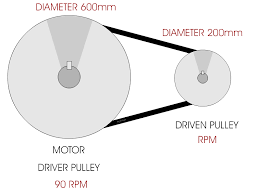The image above represents the diameter of a driven pulley.

To compute for diameter of a driven pulley, three essential parameters are needed and these parameters are Diameter of Driver Pulley (Da), r.p.m. of Driver Pulley (ra) and r.p.m. of Driven Pulley (rb).

The formula for calculating the diameter of a driven pulley:

Db = (Da x ra)/rb

Where:

Db = Diameter of Driven Pulley
Da = Diameter of Driver Pulley
ra = r.p.m. of Driver Pulley
rb = r.p.m. of Driven Pulley

Let’s solve an example;
Find the diameter of driven pulley when the diameter of driver is 3, the r.p.m of driver pulley is 8 and r.p.m of driven pulley is 22.

This implies that;

Da = Diameter of Driver Pulley = 3
ra = r.p.m. of Driver Pulley = 8
rb = r.p.m. of Driven Pulley = 22

Db = (Da x ra)/rb
Db = (3 x 8)/22
Db = 24/22
Db = 1.09

Therefore, the diameter of driven pulley is 3 m.

Nickzom Calculator – The Calculator Encyclopedia is capable of calculating the diameter of driven pulley.

To get the answer and workings of the diameter of driven pulley using the Nickzom Calculator – The Calculator Encyclopedia. First, you need to obtain the app.

You can get this app via any of these means:

To get access to the professional version via web, you need to register and subscribe for NGN 2,000 per annum to have utter access to all functionalities.
You can also try the demo version via https://www.nickzom.org/calculator

Apple (Paid) – https://itunes.apple.com/us/app/nickzom-calculator/id1331162702?mt=8
Once, you have obtained the calculator encyclopedia app, proceed to the Calculator Map, then click on Materials and Metallurgical under Engineering.Now, Click on Ball Mill Sizing under Materials and MetallurgicalNow, Click on Pulley Size under Ball Mill Sizing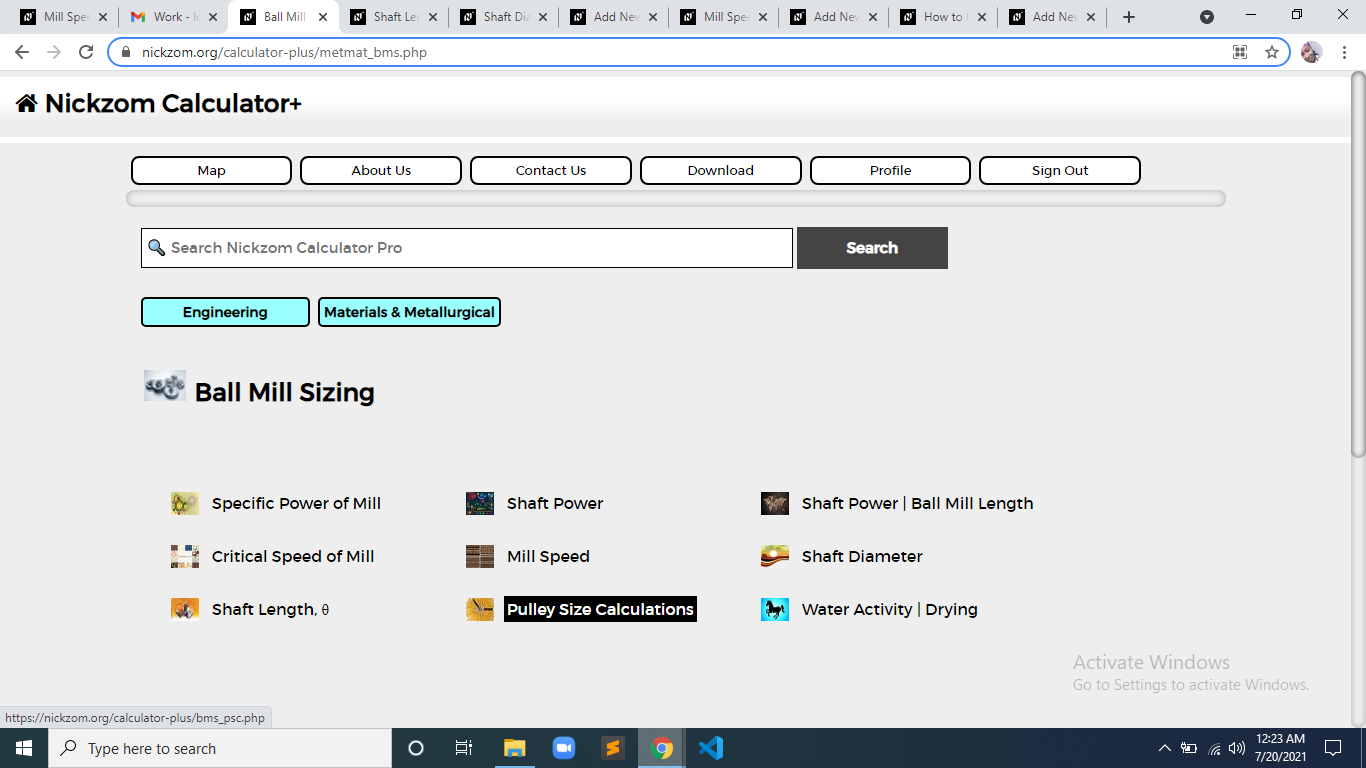Now, Click on Diameter of Driven Pulley under Pulley Size Calculations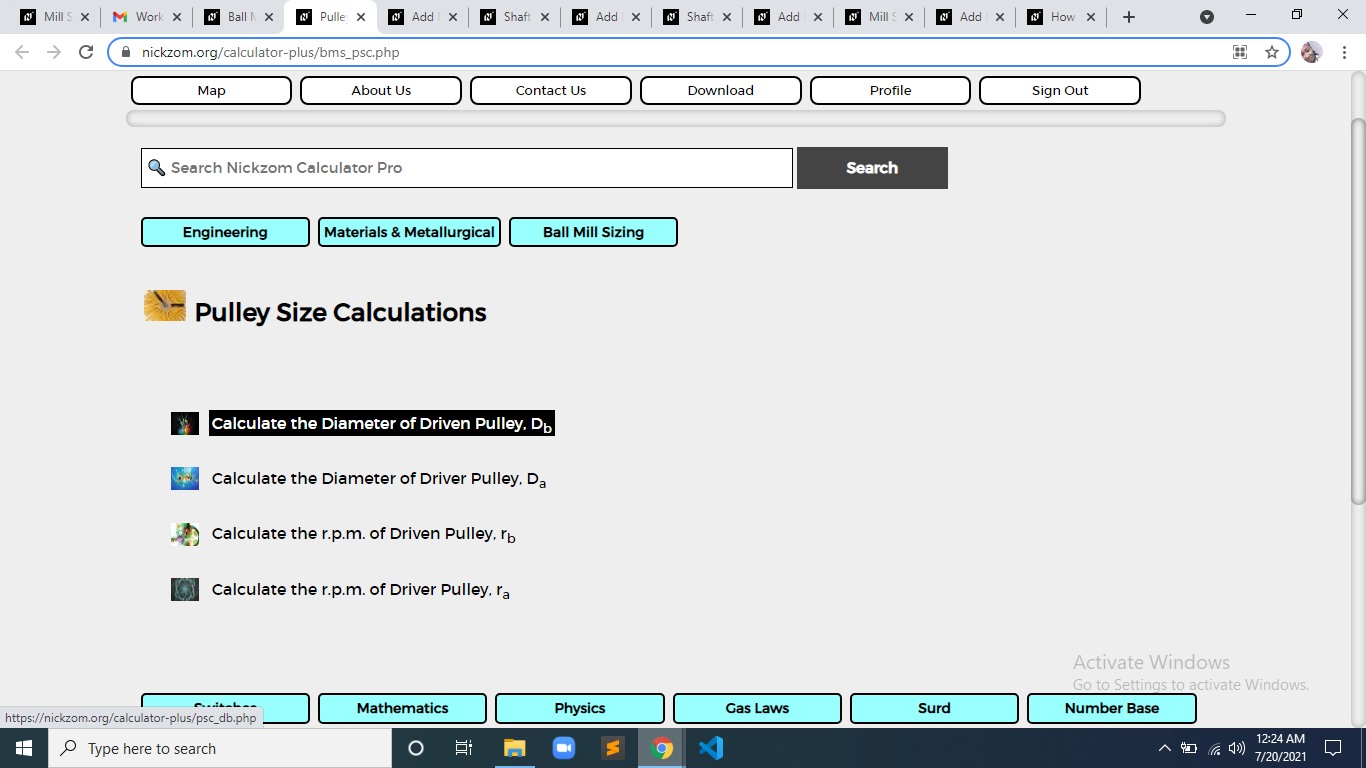The screenshot below displays the page or activity to enter your values, to get the answer for the diameter of driven pulley according to the respective parameters which is the Diameter of Driver Pulley (Da), r.p.m. of Driver Pulley (ra) and r.p.m. of Driven Pulley (rb).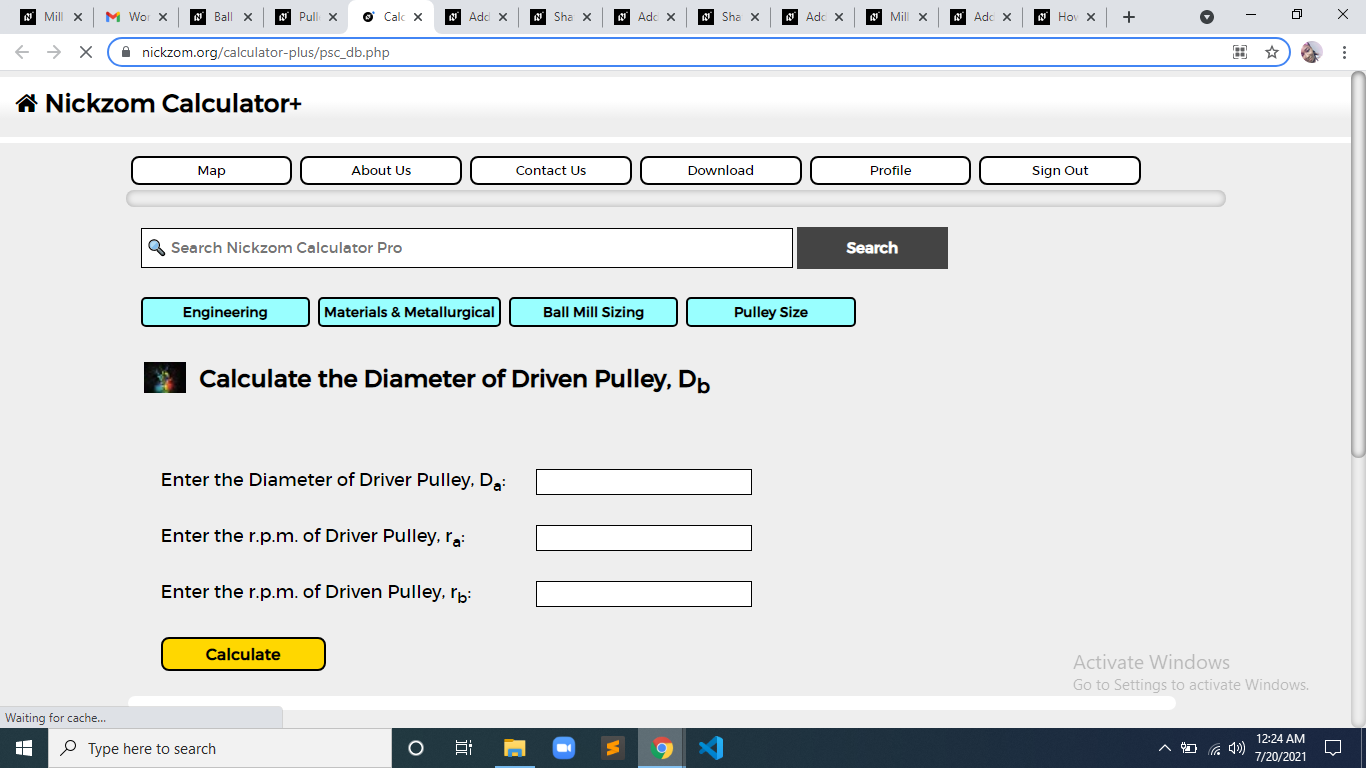Now, enter the value appropriately and accordingly for the parameter as required by the Diameter of Driver Pulley (Da) is 3, r.p.m. of Driver Pulley (rais 8 and r.p.m. of Driven Pulley (rb) is 22.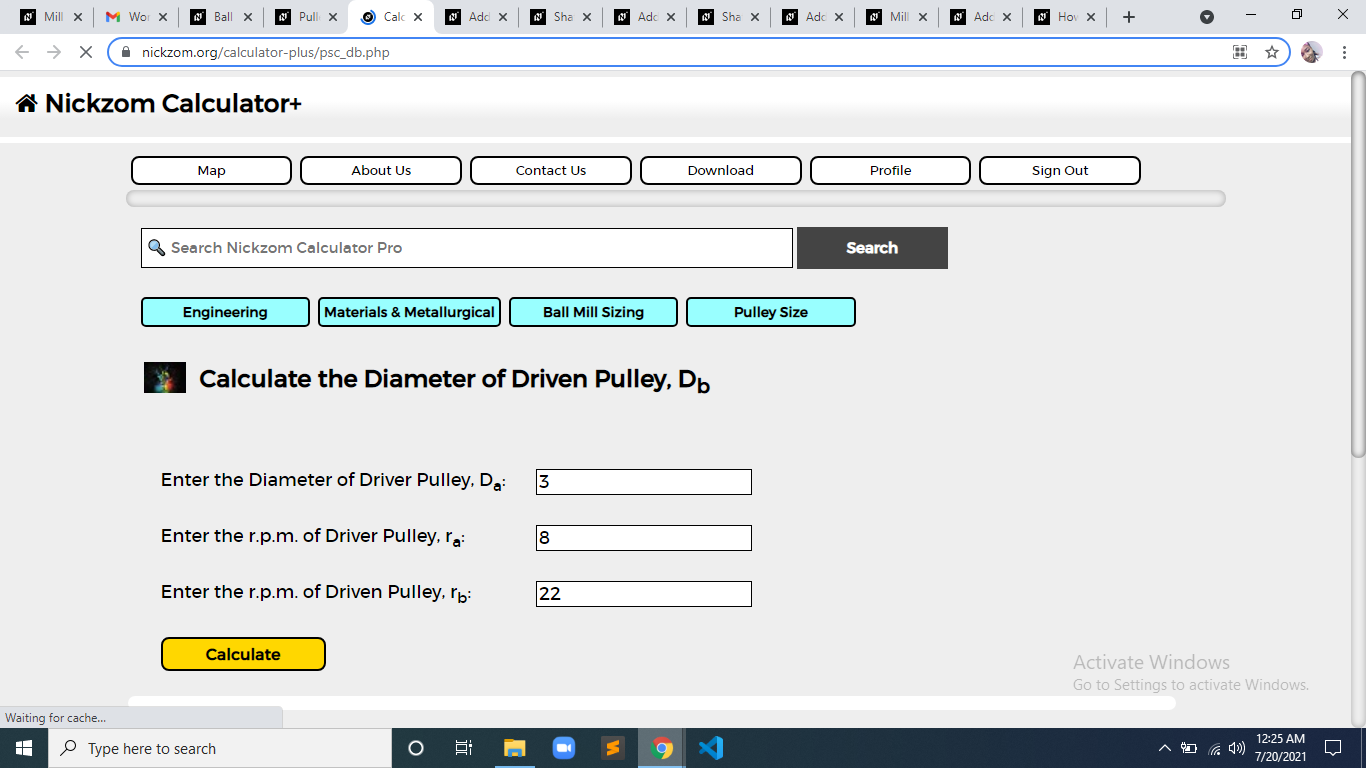Finally, Click on Calculate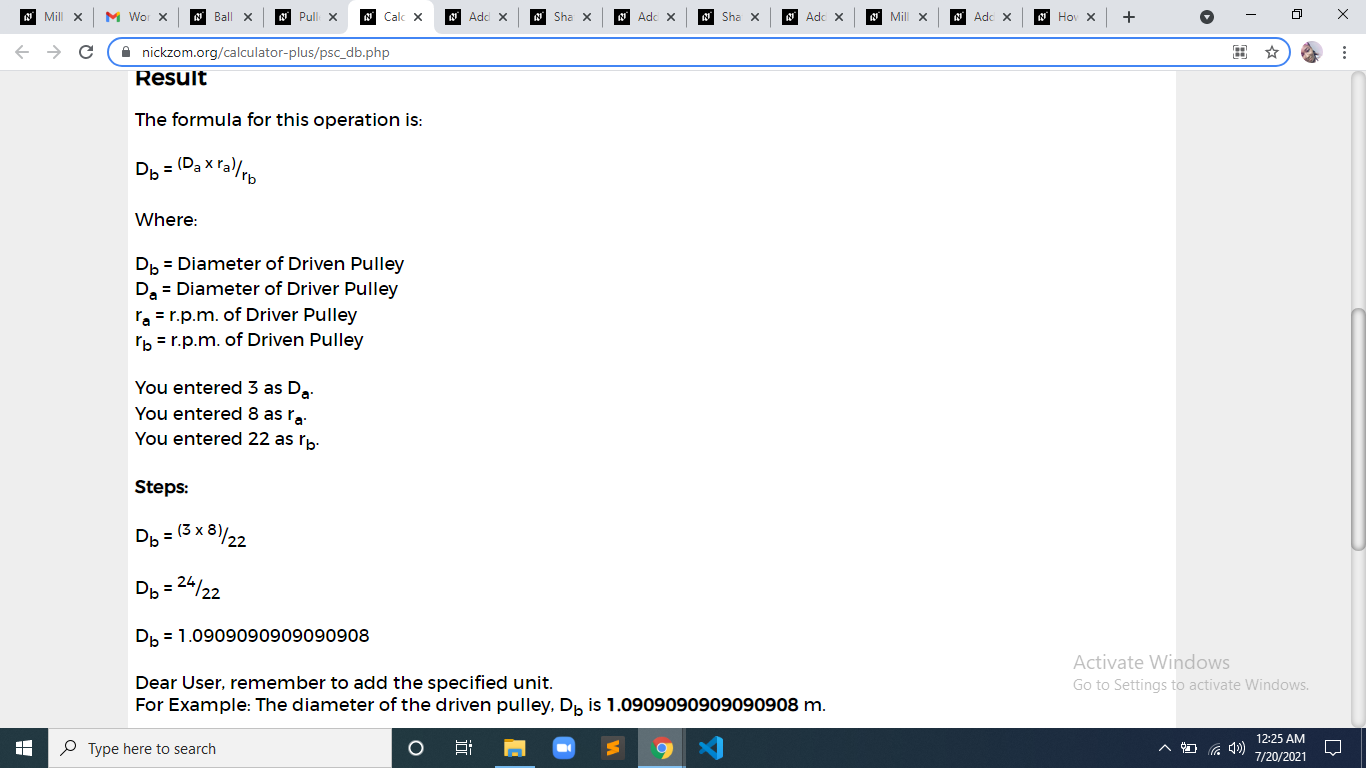As you can see from the screenshot above, Nickzom Calculator– The Calculator Encyclopedia solves for the diameter of driven pulley and presents the formula, workings and steps too.